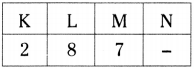AP State Syllabus AP Board 9th Class Physical Science Important Questions Chapter 5 What is inside the Atom?

## AP State Syllabus 9th Class Physical Science Important Questions 5th Lesson What is inside the Atom?

### 9th Class Physical Science 5th Lesson What is inside the Atom? 1 Mark Important Questions and Answers

Question 1.
Write any two limitations of Rutherford’s atomic model.

• The revolving electron would lose energy continuously and get directed towards positively charged nucleus and eventually crash into the nucleus.
• If this is true, the atoms would become highly unstable and the matter would not exist, but matter exists.

Question 2.
In Rutherford’s experiment, when the alpha particles hit the foil, Rutherford expected that all the alpha particles would be deflected by small angles. Why did Rutherford expect the above observation?
Rutherford thought that positive charge is distributed throughout the atom.Question 3.
Write an application of isotopes in the field of medicine.

• The isotope of iodine is used in the treatment of goitre.
• The istope of cobalt is used in the treatment of cancer.

### 9th Class Physical Science 5th Lesson What is inside the Atom? 2 Marks Important Questions and Answers

Question 1.
What is valency? Write valency of hydrogen.

• The number of electrons present in outer most orbit of an atom is called its valency.
• Valency of hydrogen is 1.

Question 2.
Observe the table given below.

 Shell Shell No Maximum number of electrons in a shell K 1 2(1)2 = 2 L 2 2(2)2 = 8 M 3 2 (3)2 = 18 N 4 2(4)2 = 32

i) Which Shell has highest number of electrons?
ii) Write the general form of the formula to find maximum number of electrons in each shell?
i) 0 Shell ‘N’.
ii) 2n2 (n = 1, 2, 3, ……..)Question 3.
Convert 36g of water into mole?
Water molecular formula = H2O
At.wt. of water = (2 × 1) + 16 = 18
1 mole, water = 18 gr.
36g. of water = $$\frac{36}{18}$$ = 2 moles

### 9th Class Physical Science 5th Lesson What is inside the Atom? 4 Marks Important Questions and Answers

Question 1.
Fill the blanks in the table using the given information. (Isotopes are not included).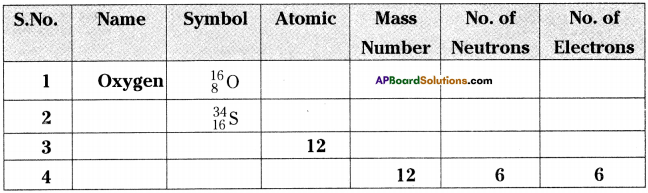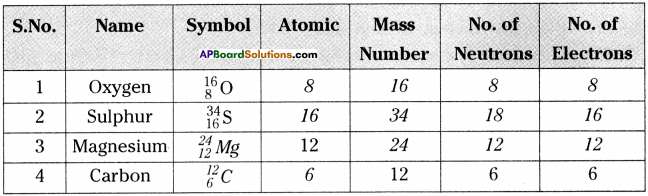Question 2.
Fill in the missing information in the table given below.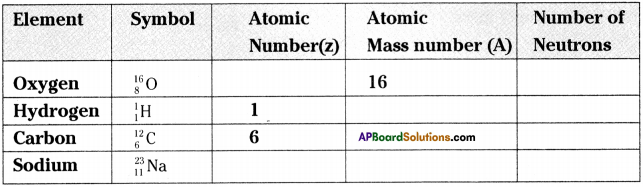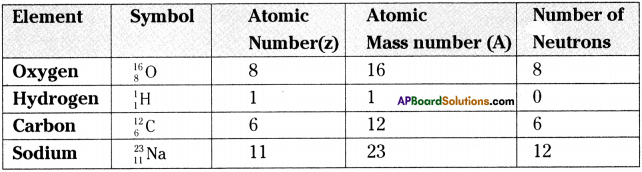Question 3.
Draw the figures showing arrangement of electrons for the given elements.
1. Helium, Oxygen, Argon.
1) Helium
2) Oxygen
3) Argon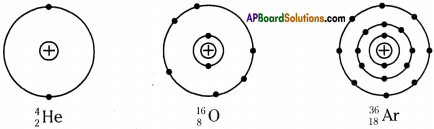2. How many neutrons are present in the nucleus of Sodium?
Sodium = 2311Na
Neutrons = 23-11 = 12

Question 4.
a) Draw neat diagrams indicating the nucleus and arragement of electrons in different shell for the following elements?
i) Helium
ii) Carbon
iii) Argon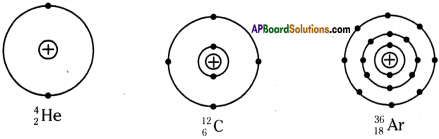b) Which of the above element is unstable? Why?
Carbon is unstable. The nudes of carbon-14 atoms are unstable because they have too many neutrons relative to protons, so they gradually decay.

### 9th Class Physical Science 5th Lesson What is inside the Atom? Important Questions and Answers

9th Class Physical Science 5th Lesson What is inside the Atom? 1 Mark Important Questions and Answers

Question 1.
Write the names of isotopes of Hydrogen.
Hydrogen, deuterium, tritium.

Question 2.
Which element has maximum number of isotopes?
Cesium and Helium are the elements having maximum number of isotopes.

Question 3.
What is maximum number of electrons present in M-shell?
Maximum number of electrons present in M-shell is 2 × 32 = 2 × 9 = 18.Question 4.
Which atom doesn’t contain neutron in its nuclear?
Hydrogen.

Question 5.
Show the arrangement of electrons in phosphorus through a diagram.
Atomic number of phosphorus is 15.
Distribution of electrons : 2, 8, 5.Question 6.
Who proposed rules for electron distribution in an atom?
Bohr and Bury.

Question 7.
What is the first rule of Bohr and Bury for electron distribution in an atom?
The maximum number of electrons present in a shell is given by the formula 2n², where ‘n’ is the shell number, which takes values 1, 2, 3, 4,

Question 8.
Write the second principle of Bohr – Bury.
Each energy level or electron shell is further divided into sub-shells. The maximum number of electrons that can be accommodated in each shell is 8.Question 9.
Write the third law of Bohr – Bury.
Electrons cannot be filled in a given shell unless the inner shells are completely filled i.e., shells are filled in step-wise manner.

Question 10.
What is an octet?
An outermost shell which has 8 electrons is said to possess an octet.

Question 11.
Define atomic number.
Atomic number :
Atomic number is the number of protons in the nucleus of an atom, denoted by ‘Z’.

Question 12.
Define atomic mass number.
Atomic mass number:
Atomic mass number is the number of protons plus the number of neutrons, denoted by A.
∵ A = Z + NQuestion 13.
On the basis of Thomson’s model of an atom, explain how the atom is neutral as a whole.
The negative and positive charges are equal in magnitude are present in atom. So, the atom as a whole is electrically neutral as whole according to Thomson model.

Question 14.
On the basis of Rutherford model of an atom, which sub-atomic particle is present in the nuclear of an atom?
α – particles are repelled by the nucleus. So it contains positive charged particle that is proton.

9th Class Physical Science 5th Lesson What is inside the Atom? 2 Marks Important Questions and Answers

Question 1.
What are the postulates of Thomson’s model of the atom?
J.J. Thomson proposed a model of atom in 1898. The main postulates are1. An atom is considered to be a sphere of uniform positive charge and electrons are embedded into it.
2. The total mass of the atom is considered to be uniformly distributed throughout the atom.
3. The negative and the positive charges are supposed to cancel out the atom as a whole is electrically neutral.

This model is commonly known as plum pudding model or watermelon model.

Question 2.
What are the features of Rutherford’s model of atom?
In 1909, Rutherford proposed a model of atom based on the alpha particle scattering experiment. The main features are

1. All the positively charged material in an atom formed a small dense centre, called the nucleus of the atom. The electrons were not a part of nucleus.
2. The negatively charged electrons revolve around the nucleus in well-defined orbits.
3. The size of the nucleus is very small as compared to the size of the atom.

This model is known as planetary model because the electrons revolve around the nucleus as planets revolve around the sun.

This model failed to explain the stability of atom.

Question 3.
Write the rules proposed by Bohr – Bury for electron distribution.
Bohr-Bury proposed the following rules for electron distribution.
Rule – 1 :
The maximum number of electrons that can be accommodated in each shell is given by a formula 2n2. Where n is the shell number, which takes the values 1, 2, 3,….
Ex : For K shell, n = 1

∴ Maximum number of electrons in K shell = 2(1)2 = 2 × 1=2

Rule – 2 : Each energy level or electron shell is further divided into subshell. The maximum number of electrons that can be accommodated in each subshell is 8.

Rule – 3: Electron cannot be filled in a given shell unless the inner shells are completely filled.Question 4.
Explain the distribution of electrons in oxygen atom using Bohr-Bury principle.

1. Atomic number of oxygen is 8.
2. Hence it has 8 protons and 8 electrons.

Step -1 :
The K-shell can accommodate maximum 2 electrons, so the first 2 electrons fill the shell of n = 1.

Step – 2 :
The other 6 electrons will fill the higher shell n = 2 or the L-shell.
Step – 3 :
Then the electronic structure for oxygen atom is 2, 6.

Question 5.
Fluorine atom contains 7 electrons in the outermost shell. But its valency is ‘1’. Explain.

• Valency is the number of electrons present in the outer most orbit of an atom.
• The distribution of electrons in fluorine (atomic number 9) is 2, 7.
• Hence the valency of fluorine could be 7.
• But it is easier to fluorine to gain one electron for becoming octet.
• Hence its valency is determined by subtracting seven electrons from 8 and which gives you a valency ‘1’ for fluorine.

Question 6.
What is the importance of valency?
1) An atom with 8 electrons or an octet in their outer most shell is chemically stable or does not combine other atoms.
Ex : Ne, Ar, etc.

2) An atom with duplet or 2 electrons in its outer most shell is also more stable when there is only one shell present in it.
Ex : He

3) Atoms of an element thus react with other atoms. So as to achieve an octet in their shell.

4) When elements reacts to form compounds, their atoms must be combining in such a way that they can attain the stable electron distribution of noble gases or inert gases.Question 7.
How can an atom achieve octet?
An atom can achieve an octet by two ways.

1. One by transfer of electrons.
2. Other by sharing of electrons.
3. Both the processes results in the formation of bonds between atoms.

Question 8.
Explain the notation : $${ }_{9}^{19} \mathrm{F}$$

• F is the symbol of element (Fluorine).
• 9 is the atomic number.
• 19 is the mass number.
• Hence fluorine has 9 protons and (19 – 9 = 10) 10 neutrons in its nucleus.
• 9 electrons are revolving around the nucleus.

Question 9.
Define isotope and give two examples.
Isotope :
The atoms of the same element which have the same number of protons but have different number of neutrons are called isotopes.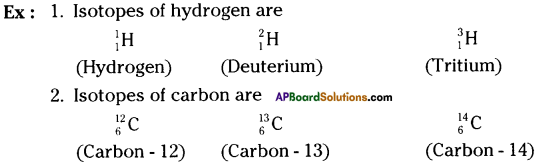Question 10.
“Sulphur shows multiple valency.” Explain this.
a) Atomic number of sulphur is 16.
b) The distribution of electrons is 2, 8, 6.
c) Hence the valency should be ’6′.
d) But it is easier to sulphur to gain 2 electrons than loosing 6 electrons to become octet. Hence its valency would become 2.
e) So, sulphur shows multiple valency, i.e., ‘2’ or ‘6’.

Question 11.
Show the arrangement of electrons in first 18 elements schematically.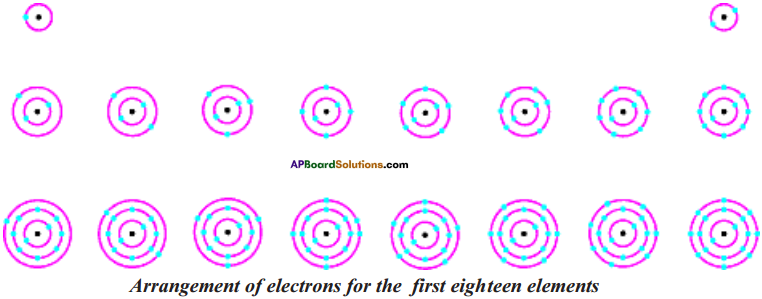Question 12.
What are the applications of isotopes in our daily life?
Applications of isotopes :

1. Some isotopes are used for solving chemical and medical mysteries.
2. Isotopes are also commonly used in the laboratory to investigate the steps of a chemical reaction.
3. The isotope of uranium is used as a fuel in nuclear reactors.
4. The isotope of iodine is used in the treatment of goitre.
5. The isotope of cobalt is used in the treatment of cancer.

Question 13.
If an atom contains one electron and one proton, will it carry any charge or not?
It will not carry any charge because proton is positively charged particle and electron is negatively charged particle. So they would neutralise each other.

Question 14.
What do you think would be the observation if the a – particle scattering experiment is carried out using a foil of metal other than gold?
Gold has highest malleability and ductility. Extremely very thin foil can be prepared by using Gold, which is not possible with other metals. So, we will not get same type of results using other metals.Question 15.
Helium atom has an atomic mass of 4u and two protons in its nucleus. How many neutrons does it have?
Atomic mass due to protons = 2 × 1 = 2 u
Neutrons have almost same mass as protons.
Mass of neutrons = 4 – 2 = 2u
∴ Number of neutrons = 2

Question 16.
Write the distribution of electrons in carbon and sodium atoms.
Distribution of electrons in carbon is 2, 4.
Distribution of electrons in sodium is 2, 8, 1.

Question 17.
If K and L shells of an atom are full, then what would be the total number of electrons in the atom?
Number of electrons present in K shell = 2(1)² = 2 × 1=2
Number of electrons present in L shell = 2(2)² = 2 × 4 = 8
Total number of electrons in the atom = 2 + 8 = 10

Question 18.
If number of electrons in an atom is 8 and number of protons is also 8, then
i) what is the atomic number of atom?
ii) what is the charge on the atom?
i) The atomic number of atom is 8.
ii) The electronic configuration of atom is 2, 6. By gaining two electrons it will get nearest inert gas configuration Neon. So, the charge on the atom is – 2.Question 19.
Chlorine occurs in two isotopic forms that is $${ }_{17}^{35} \mathrm{Cl}$$ and $${ }_{17}^{37} \mathrm{Cl}$$. The percentage of these forms are 75% and 25% respectively. Find atomic weight of chlorine atom?
The atomic mass of an element is taken as an average mass of all the naturally occurring atoms of the sample element.
The average atomic mass of chlorine atom on the bases of above data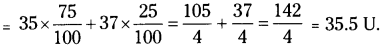Question 20.
For the symbol H, D and T tabulate three sub atomic particles found in each of them.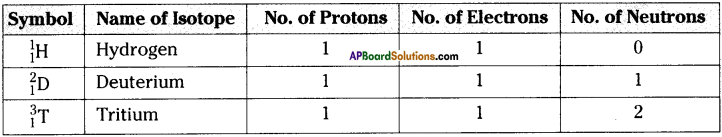Question 21.
Na+ has completely filled K and L shells. Explain.
Electron configuration of Na+ is 2, 8.
So, sodium has completed filled K and L shells because the maximum number of electrons filled in K and L shells are 2 and 8 electrons respectively.

Question 22.
If Bromine atom is available in the form of say, two isotopes $${ }_{35}^{79} \mathrm{Br}$$(49.7%) and $${ }_{35}^{81} \mathrm{Br}$$ (50.3%), calculate the average atomic mass of Bromine atom.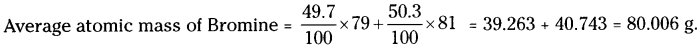Question 23.
The average atomic mass of sample of an element X is 16.2 u. What are the percentages of isotopes $${ }_{8}^{16} \mathrm{X}$$ and $${ }_{8}^{18} \mathrm{X}$$?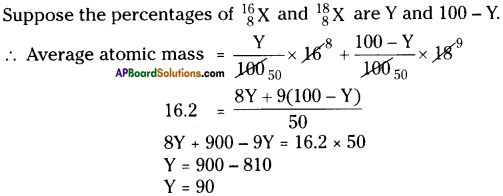∴ The percentage of first isotope is 90 and percentage of second isotope is 10.

Question 24.
If Z = 3, what would be the valency of the elements? Also, name the element?
Electronic configuration of element is 2, 1 and the element is Lithium.
The element will get nearest inert gas Helium configuration by loosing one electron. So its valency is 1.

Question 25.
Composition of nuclei of two atomic species X and Y are given as under.

 X Y Protons 6 6 Neutrons 6 8

Give the mass number of X and Y. What is the relation between the two species?
Mass number of X = 6 + 6 = 12
Mass number of Y = 6 + 8 = 14
So these two species have same atomic number (same number of protons) and different mass numbers. Therefore these two species are isotopes.

9th Class Physical Science 5th Lesson What is inside the Atom? 4 Marks Important Questions and Answers

Question 1.
How did the idea of sub-atomic particles evolve?

• According to Dalton, atom is indivisible.
• But Michael Faraday’s experiments on electrolysis proved that atoms were acquiring negative charge during electrolysis.
• This is contradiction to Dalton’s theory.
• This lead to an idea that there must exist some tiny particles in atom which are responsible for atom to behave sometimes as charged particles.
• As atom is considered as electrically neutral, it probably had equal number of positive and negative constituents to maintain electrical neutrality.
• This gave scope to think about sub-atomic particles.Question 2.
How do we determine the atomic mass of an element with its isotopes?

• In nature, most elements occur as a mixture of two or more isotopes, each isotope has a certain percentage of natural occurrence.
• The atomic mass of an element is taken as an average mass of all the naturally occuring atoms of the sample element.
Ex : Let us calculate the atomic mass of chlorine.
• Isotope of Cl occurs in nature, in two isotopic forms, with masses 35 units and 37 units.
• The isotope with mass 35 is present in 75% in nature.
• The isotope with mass 37 is present in 25% in nature.
• The average mass of chlorine is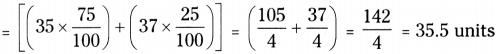Question 3.
Describe the Rutherford’s alpha particle scattering experiment. What are the conclu¬sions of this experiment.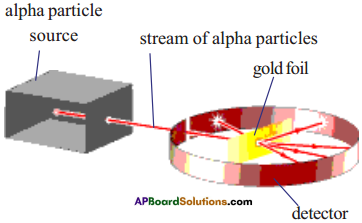Rutherford conducted alpha particle scattering experiment in 1909 to study the atom.

1. The stream of alpha particles from a source having considerable energy is directed towards a very thin gold foil.
2. The gold foil was placed inside a detector in such a way that the detector would show a flash of light when an alpha particle struck it.
3. The entire arrangement was kept in a vacuum chamber.
4. Rutherford did not expect to see large deflections of alpha particles based on Thomson’s model.
5. But Rutherford observed the deflection of alpha particles through very large angles and a few alpha particles were reflected right back.
6. From this observation, Rutherford concluded as follows.Conclusions :

1. Most of the space inside the atom is empty.
2. All the positive charge must be concentrated in a very small space within the atom and he named it as ‘nucleus’.

Question 4.
What information do you know from $${ }_{17}^{35} \mathrm{X}$$?
Given that $${ }_{17}^{35} \mathrm{X}$$